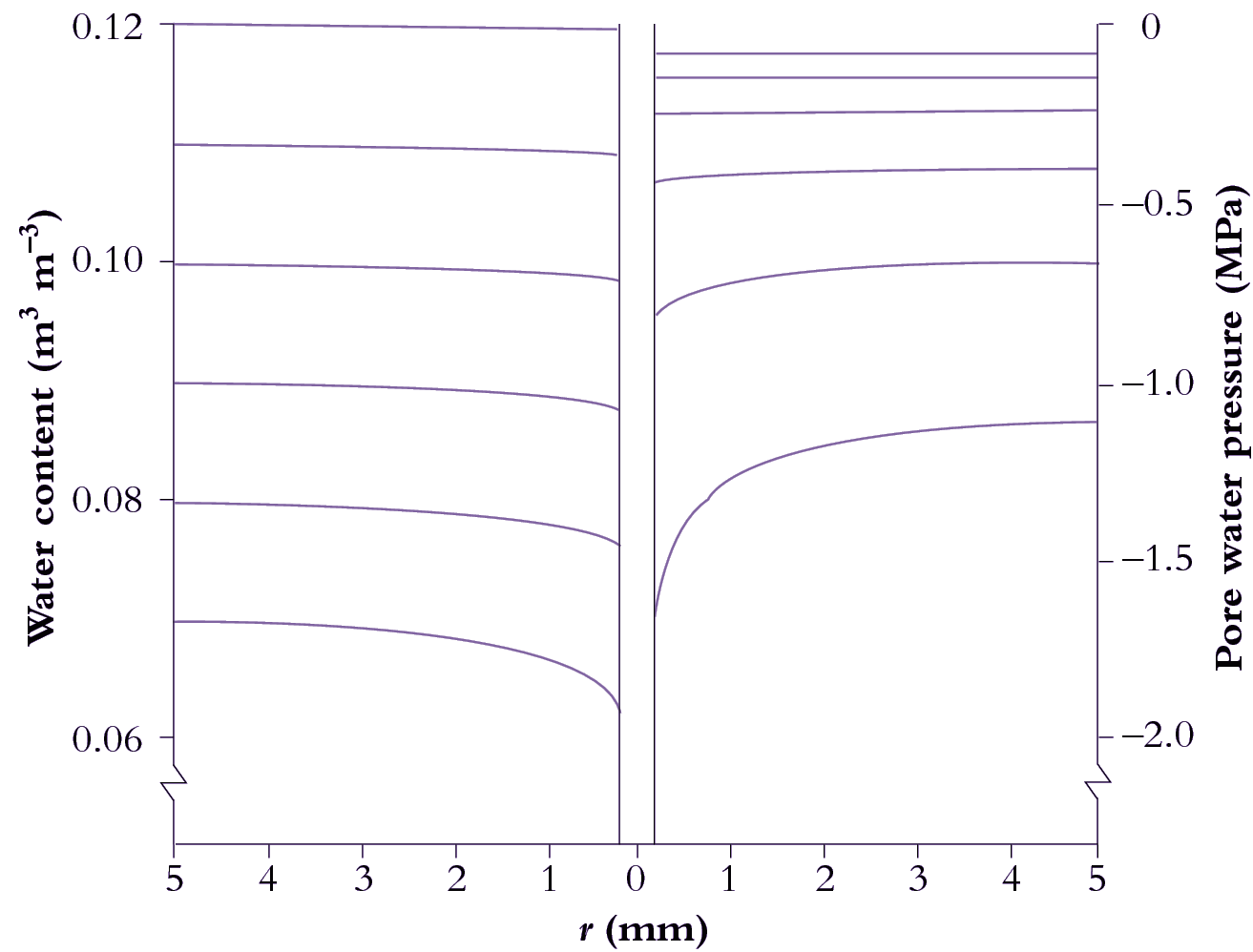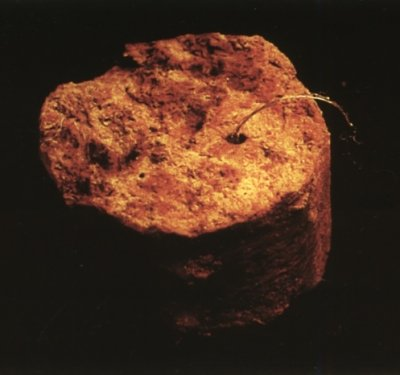# 3.4.3 - Uptake of water by roots

Flow of water through soil is induced by gradients in hydrostatic pressure, P. The rate of flow, F (m s–1), depends on both the gradient in P and on hydraulic conductivity, K  (m2 MPa–1 s–1), of the soil:

$F=K \frac{dP}{dx} \tag{8}$

where x (m) is distance. This equation is Darcy’s Law. Conductivity, K, varies enormously, by about a thousand-fold, over the range of available water content. The reason for this large range is that water flows much more easily in a large pore than in a small one. As explained earlier (Section 3.1), the flow rate varies according to Poiseuille’s Law — the flow in capillary tubes depends on the fourth power of the diameter. As the soil dries, the large pores are empty and water is drawn from smaller and smaller pores.

Water removed by transpiration results in drier soil around roots compared with bulk soil. As soil dries near the root surface, water flows radially from bulk soil to replenish it. Calculated distributions of water content and pore water pressure with radial distance from an absorbing root predict a pronounced increase in suction adjacent to the root.

### (a) Theory of water uptake

Roots are cylindrical, so the flow of water to them is radial. This has considerable consequences: the flow lines converge as they approach the root, which requires increasing gradients of pressure in the soil water to keep the water flowing (Figure 3.41).

## 3.0-Ch-Fig-3.41.pngFigure 3.41 Calculated distributions of volumetric soil water content (left) and pressure in water-filled pores (right) as functions of distance from a model root. Pressures become more negative over time, indicating increasing suction. Horizontal lines denote water status on each of six successive days (day 1 is uppermost). The steepening of curves at later times reflects how transport of water from bulk soil to the root surface becomes increasingly difficult as the soil dries. r = distance from central axis of root.

A radial flow of water from the bulk soil towards roots of transpiring plants is generated by suction at the root surface. However, because K decreases with falling water content, there is a limit to how fast roots can extract water from soil. Once this limit has been reached, increasing suction by roots simply steepens the gradient in P to match the fall in K close to root surfaces so that the product of the two (Equation 3.1) remains the same.

Although soil water is driven by gradients of pressure, it is necessary when water content is changing to describe this flow in terms of gradients in volumetric water content, θ (m3 m–3). The coefﬁcient relating flow rate to the gradient in water content is known as diffusivity, D (m2 s–1), and the appropriate equation is formally analogous to Fick’s First Law of diffusion:

$F=D \frac{d\theta}{dx} \tag{9}$

This equation can be elaborated to allow for the cylindrical flow, and then solved to derive an approximate relationship for the uptake of water by a population of roots:

$Q \approx 2D*\Delta \theta * L \tag{10}$

where Q is the flow rate of water through the soil, (m3 m–3 s–1), now expressed as the overall rate of change of θ in the sample soil, and L is the rooting density, average length of absorbing root per unit volume of soil (m m–3).

Like K, D varies with the soil water content, although not so widely.  Laboratory measurements of  D, which are so far the only ones that have been made with some accuracy, show that D is about 10-7 m2s-1 when the soil is fairly wet, and falls to about 10-9 m2s-1 once the soil has dried far enough for the soil water suction to exceed about 300 kPa.  Where D is of the order of 10-9 m2 s-1 one can calculate from eqn 3.3 that wherever the density of roots exceeds about 1 cm per cm3 of soil (104 m m-3) the flow of water through the soil is not likely to limit uptake until there is almost no available water left in the soil. But where there is less than about 1 mm of root per cm3 (103 m m-3) of soil, uptake is likely to be sufficiently limited by flow through the soil that it would take several days for the roots to extract most of the available water.

## 3.0-Ch-Fig-3.42-v2.jpegFigure 3.42 Sample of dense subsoil containing a biopore occupied by a root. Such biopores are passages for roots through otherwise almost impenetrably dense soil.

Many experiments have been done to explore this theory, with mixed results.  Most have involved growing plants in repacked soil in controlled laboratory conditions. Some have confirmed the theory, many have not. The most likely reasons for the discrepancies is that interfacial effects - at the junction between the surface of the root and the soil - come into play.  These are discussed in the next section.

In field soil, the concentration of roots in the topsoil is usually so high that the local rate of uptake of water is unlikely to be ever limited by the properties of the soil.  It is in the subsoil that the rooting density drops to a level at which flow through the soil may limit the rate of uptake. Further, the rate of uptake is often much lower than what the simple theory would predict, even when the often sparse rooting density is taking into account.

In subsoil, there are other possible reason for the discrepancy between theory and observation.  One is that, in contrast to disturbed soil, either in ploughed topsoil or repacked in lab experiments, roots in the subsoil do not ramify more or less randomly through the soil. Subsoils are usually dense and difficult to penetrate.  Roots grow predominantly in pre-existing fissures or in continuous large pores, biopores, made by previous roots or soil fauna (Figure 3.42).

A second reason is that it may be wrong to extrapolate laboratory measurements of D in repacked soil to undisturbed soil in the field. The undisturbed structure of the soil may inhibit the flow of water.  For example, the formation of aggregates of particles in the soil often results in particles of clay (which are usually in the form of small plates) becoming oriented parallel to the surface. Such orientation is likely to increase greatly the flow path for the water, but so far no reliable measurements of D have been yet made on undisturbed subsoil.

In summary, the dense root systems common in topsoils extract water effectively from surface soil layers. Extracting water from subsoil layers is more difﬁcult. Australian subsoils are typically inhospitable to roots. They are dense, have a large resistance to penetration. They are often sodic, that is, sodium dominates the exchange complexes on soil particles, altering the soil structure so that it sets like concrete when dry, and becomes impermeable to water when wet. Moreover, subsoils can be acutely deﬁcient in some nutrients that are required locally by roots. Native vegetation overcomes these difﬁculties by forming deep biopores in the subsoil. For example, roots of jarrah trees can create and maintain a path to water deep in the subsoil, possibly even as far as a water table 20 m below the surface.